# Pitch Tracking Using Multiple Pitch Estimations and HMM

This example shows how to perform pitch tracking using multiple pitch estimations, octave and median smoothing, and a hidden Markov model (HMM).

### Introduction

Pitch detection is a fundamental building block in speech processing, speech coding, and music information retrieval (MIR). In speech and speaker recognition, pitch is used as a feature in a machine learning system. For call centers, pitch is used to indicate the emotional state and gender of customers. In speech therapy, pitch is used to indicate and analyze pathologies and diagnose physical defects. In MIR, pitch is used to categorize music, for query-by-humming systems, and as a primary feature in song identification systems.

Pitch detection for clean speech is mostly considered a solved problem. Pitch detection with noise and multi-pitch tracking remain difficult problems. There are many algorithms that have been extensively reported on in the literature with known trade-offs between computational cost and robustness to different types of noise.

Usually, a pitch detection algorithm (PDA) estimates the pitch for a given time instant. The pitch estimate is then validated or corrected within a pitch tracking system. Pitch tracking systems enforce continuity of pitch estimates over time.

This example provides an example function, `HelperPitchTracker`, which implements a pitch tracking system. The example walks through the algorithm implemented by the `HelperPitchTracker` function.

### Problem Summary

Load an audio file and corresponding reference pitch for the audio file. The reference pitch is reported every 10 ms and was determined as an average of several third-party algorithms on the clean speech file. Regions without voiced speech are represented as `nan`.

```[x,fs] = audioread("Counting-16-44p1-mono-15secs.wav"); load TruePitch.mat truePitch```

Use the `pitch` function to estimate the pitch of the audio over time.

`[f0,locs] = pitch(x,fs);`

Two metrics are commonly reported when defining pitch error: gross pitch error (GPE) and voicing decision error (VDE). Because the pitch algorithms in this example do not provide a voicing decision, only GPE is reported. In this example, GPE is calculated as the percent of pitch estimates outside $±10%$ of the reference pitch over the span of the voiced segments.

Calculate the GPE for regions of speech and plot the results. Listen to the clean audio signal.

```isVoiced = ~isnan(truePitch); f0(~isVoiced) = nan; p = 0.1; GPE = mean(abs(f0(isVoiced)-truePitch(isVoiced)) > truePitch(isVoiced).*p).*100; t = (0:length(x)-1)/fs; t0 = (locs-1)/fs; sound(x,fs) figure(1) tiledlayout(2,1) nexttile plot(t,x) ylabel("Amplitude") title("Pitch Estimation of Clean Signal") nexttile plot(t0,[truePitch,f0]) legend("Reference","Estimate",Location="northwest") ylabel("F0 (Hz)") xlabel("Time (s)") title("GPE = " + round(GPE,2) + " (%)")```Mix the speech signal with noise at $-5$ dB SNR.

Use the `pitch` function on the noisy audio to estimate the pitch over time. Calculate the GPE for regions of voiced speech and plot the results. Listen to the noisy audio signal.

```desiredSNR = -5; x = mixSNR(x,rand(size(x)),desiredSNR); [f0,locs] = pitch(x,fs); f0(~isVoiced) = nan; GPE = mean(abs(f0(isVoiced) - truePitch(isVoiced)) > truePitch(isVoiced).*p).*100; sound(x,fs) figure(2) tiledlayout(2,1) nexttile plot(t,x) ylabel("Amplitude") title("Pitch Estimation of Noisy Signal") nexttile plot(t0,[truePitch,f0]) legend("Reference","Estimate",Location="northwest") ylabel("F0 (Hz)") xlabel("Time (s)") title("GPE = " + GPE + " (%)")```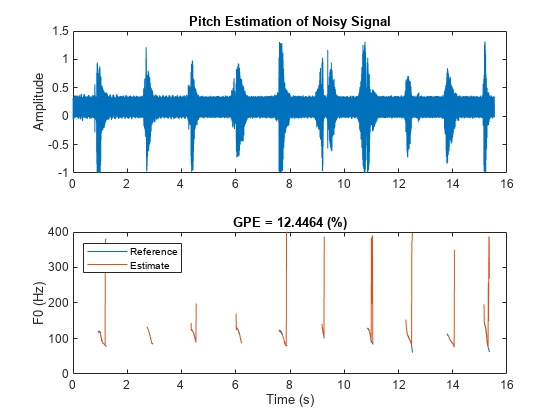This example shows how to improve the pitch estimation of noisy speech signals using multiple pitch candidate generation, octave-smoothing, median-smoothing, and an HMM.

The algorithm described in this example is implemented in the example function `HelperPitchTracker`. To learn about the `HelperPitchTracker` function, enter `help HelperPitchTracker` at the command line.

`help HelperPitchTracker`
``` HelperPitchTracker Track the fundamental frequency of audio signal f0 = HelperPitchTracker(audioIn,fs) returns an estimate of the fundamental frequency contour for the audio input. Columns of the input are treated as individual channels. The HelperPitchTracker function uses multiple pitch detection algorithms to generate pitch candidates, and uses octave smoothing and a Hidden Markov Model to return an estimate of the fundamental frequency. f0 = HelperPitchTracker(...,'HopLength',HOPLENGTH) specifies the number of samples in each hop. The pitch estimate is updated every hop. Specify HOPLENGTH as a scalar integer. If unspecified, HOPLENGTH defaults to round(0.01*fs). f0 = HelperPitchTracker(...,'OctaveSmoothing',TF) specifies whether or not to apply octave smoothing. Specify as true or false. If unspecified, TF defaults to true. f0 = HelperPitchTracker(...,'EmissionMatrix',EMISSIONMATRIX) specifies the emission matrix used for the HMM during the forward pass. The default emission matrix was trained on the Pitch Tracking Database from Graz University of Technology. The database consists of 4720 speech segments with corresponding pitch trajectories derived from laryngograph signals. The emission matrix corresponds to the probability that a speaker leaves one pitch state to another, in the range [50, 400] Hz. Specify the emission matrix such that rows correspond to the current state, columns correspond to the possible future state, and the values of the matrix correspond to the probability of moving from the current state to the future state. If you specify your own emission matrix, specify its corresponding EMISSIONMATRIXRANGE. EMISSIONMATRIX must be a real N-by-N matrix of integers. f0 = HelperPitchTracker(...,'EmissionMatrixRange',EMISSIONMATRIXRANGE) specifies how the EMISSIONMATRIX corresponds to Hz. If unspecified, EMISSIONMATRIXRANGE defaults to 50:400. [f0,loc] = HelperPitchTracker(...) returns the locations associated with each pitch decision. The locations correspond to the ceiling of the center of the analysis frames. [f0,loc,hr] = HelperPitchTracker(...) returns the harmonic ratio associated with each pitch decision. See also pitch, voiceActivityDetector ```

### Description of Pitch Tracking System

The graphic provides an overview of the pitch tracking system implemented in the example function. The following code walks through the internal workings of the `HelperPitchTracker` example function.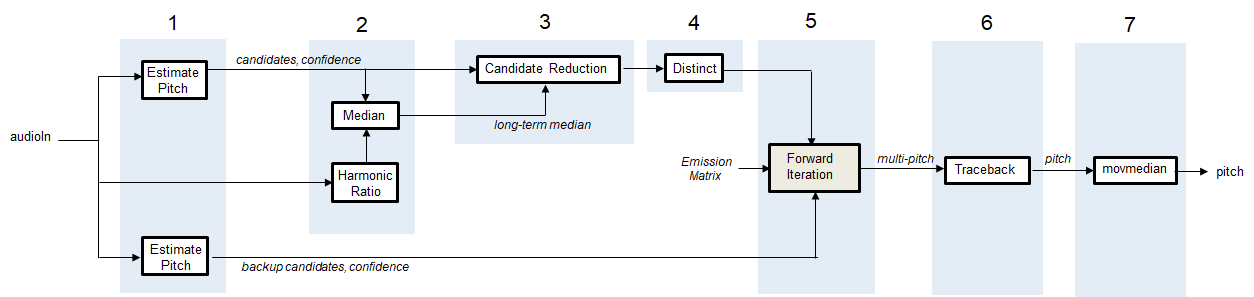### 1. Generate Multiple Pitch Candidates

In the first stage of the pitch tracking system, you generate multiple pitch candidates using multiple pitch detection algorithms. The primary pitch candidates, which are generally more accurate, are generated using algorithms based on the Summation of Residual Harmonics (SRH)  algorithm and the Pitch Estimation Filter with Amplitude Compression (PEFAC)  algorithm.

Buffer the noisy input signal into overlapped frames, and then use `audio.internal.pitch.SRH` to generate 5 pitch candidates for each hop. Also return the relative confidence of each pitch candidate. Plot the results.

```RANGE = [50,400]; HOPLENGTH = round(fs.*0.01); % Buffer into required sizes xBuff_SRH = buffer(x,round(0.025*fs),round(0.02*fs),"nodelay"); % Define pitch parameters params_SRH = struct(Method="SRH", ... Range=RANGE, ... WindowLength=round(fs*0.06), ... OverlapLength=round(fs*0.06-HOPLENGTH), ... SampleRate=fs, ... NumChannels=size(x,2), ... SamplesPerChannel=size(x,1)); multiCandidate_params_SRH = struct(NumCandidates=5,MinPeakDistance=1); % Get pitch estimate and confidence [f0_SRH,conf_SRH] = audio.internal.pitch.SRH(xBuff_SRH,x,fs, ... params_SRH, ... multiCandidate_params_SRH);```
```figure(3) tiledlayout(2,1) nexttile plot(t0,f0_SRH) ylabel("F0 Candidates (Hz)") title("Multiple Candidates from SRH Pitch Estimation") nexttile plot(t0,conf_SRH) ylabel("Relative Confidence") xlabel("Time (s)")```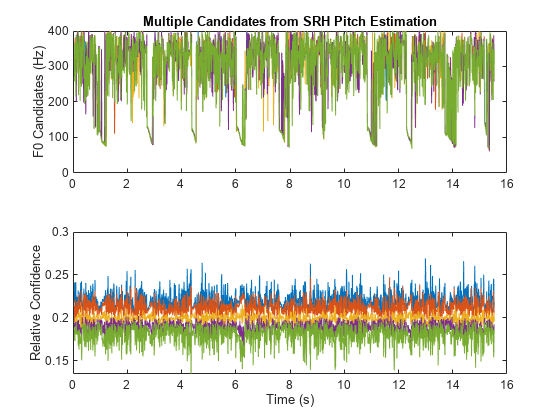Generate an additional set of primary pitch candidates and associated confidence using the PEF algorithm. Generate backup candidates and associated confidences using the normalized correlation function (NCF) algorithm and cepstrum pitch determination (CEP) algorithm. Log only the most confident estimate from the backup candidates.

```xBuff_PEF = buffer(x,round(0.06*fs),round(0.05*fs),"nodelay"); params_PEF = struct(Method="PEF", ... Range=RANGE, ... WindowLength=round(fs*0.06), ... OverlapLength=round(fs*0.06-HOPLENGTH), ... SampleRate=fs, ... NumChannels=size(x,2), ... SamplesPerChannel=size(x,1)); multiCandidate_params_PEF = struct(NumCandidates=5,MinPeakDistance=5); [f0_PEF,conf_PEF] = audio.internal.pitch.PEF(xBuff_PEF,fs, ... params_PEF, ... multiCandidate_params_PEF); xBuff_NCF = buffer(x,round(0.04*fs),round(0.03*fs),"nodelay"); xBuff_NCF = xBuff_NCF(:,2:end-1); params_NCF = struct(Method="NCF", ... Range=RANGE, ... WindowLength=round(fs*0.04), ... OverlapLength=round(fs*0.04-HOPLENGTH), ... SampleRate=fs, ... NumChannels=size(x,2), ... SamplesPerChannel=size(x,1)); multiCandidate_params_NCF = struct(NumCandidates=5,MinPeakDistance=1); f0_NCF = audio.internal.pitch.NCF(xBuff_NCF,fs, ... params_NCF, ... multiCandidate_params_NCF); xBuff_CEP = buffer(x,round(0.04*fs),round(0.03*fs),"nodelay"); xBuff_CEP = xBuff_CEP(:,2:end-1); params_CEP = struct(Method="CEP", ... Range=RANGE, ... WindowLength=round(fs*0.04), ... OverlapLength=round(fs*0.04-HOPLENGTH), ... SampleRate=fs, ... NumChannels=size(x,2), ... SamplesPerChannel=size(x,1)); multiCandidate_params_CEP = struct(NumCandidates=5,MinPeakDistance=1); f0_CEP = audio.internal.pitch.CEP(xBuff_CEP,fs, ... params_CEP, ... multiCandidate_params_CEP); BackupCandidates = [f0_NCF(:,1),f0_CEP(:,1)];```

### 2. Determine Long-Term Median

The long-term median of the pitch candidates is used to reduce the number of pitch candidates. To calculate the long-term median pitch, first calculate the harmonic ratio. Pitch estimates are only valid in regions of voiced speech, where the harmonic ratio is high.

```hr = harmonicRatio(xBuff_PEF,fs, ... Window=hamming(size(xBuff_NCF,1),"periodic"), ... OverlapLength=0); figure(4) tiledlayout(2,1) nexttile plot(t,x) ylabel("Amplitude") nexttile plot(t0,hr) ylabel("Harmonic Ratio") xlabel("Time (s)")```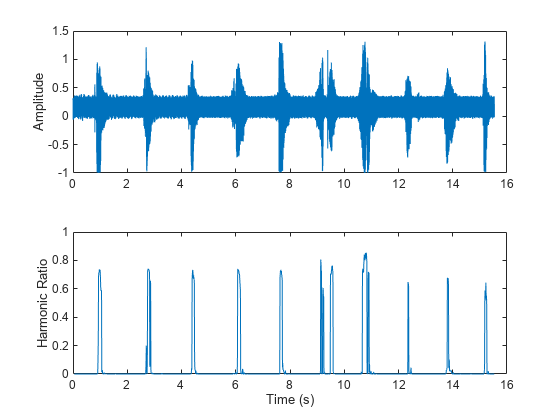Use the harmonic ratio to threshold out regions that do not include voiced speech in the long-term median calculation. After determining the long-term median, calculate lower and upper bounds for pitch candidates. In this example, the lower and upper bounds were determined empirically as 2/3 and 4/3 the median pitch. Candidates outside of these bounds are penalized in the following stage.

```idxToKeep = logical(movmedian(hr>((3/4)*max(hr)),3)); longTermMedian = median([f0_PEF(idxToKeep,1);f0_SRH(idxToKeep,1)]); lower = max((2/3)*longTermMedian,RANGE(1)); upper = min((4/3)*longTermMedian,RANGE(2)); figure(5) tiledlayout(1,1) nexttile plot(t0,[f0_PEF,f0_SRH]) hold on plot(t0,longTermMedian.*ones(size(f0_PEF,1)),"r:",LineWidth=3) plot(t0,upper.*ones(size(f0_PEF,1)),"r",LineWidth=2) plot(t0,lower.*ones(size(f0_PEF,1)),"r",LineWidth=2) hold off xlabel("Time (s)") ylabel("Frequency (Hz)") title("Long Term Median")```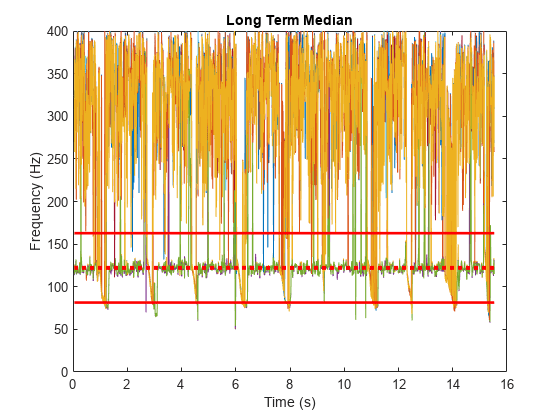### 3. Candidate Reduction

By default, candidates returned by the pitch detection algorithm are sorted in descending order of confidence. Decrease the confidence of any primary candidate outside the lower and upper bounds. Decrease the confidence by a factor of 10. Re-sort the candidates for both the PEF and SRH algorithms so they are in descending order of confidence. Concatenate the candidates, keeping only the two most confident candidates from each algorithm.

Plot the reduced candidates.

```invalid = f0_PEF>lower | f0_PEF>upper; conf_PEF(invalid) = conf_PEF(invalid)/10; [conf_PEF,order] = sort(conf_PEF,2,"descend"); for i = 1:size(f0_PEF,1) f0_PEF(i,:) = f0_PEF(i,order(i,:)); end invalid = f0_SRH>lower | f0_SRH>upper; conf_SRH(invalid) = conf_SRH(invalid)/10; [conf_SRH,order] = sort(conf_SRH,2,"descend"); for i = 1:size(f0_SRH,1) f0_SRH(i,:) = f0_SRH(i,order(i,:)); end candidates = [f0_PEF(:,1:2),f0_SRH(:,1:2)]; confidence = [conf_PEF(:,1:2),conf_SRH(:,1:2)]; figure(6) plot(t0,candidates) xlabel("Time (s)") ylabel("Frequency (Hz)") title("Reduced Candidates")```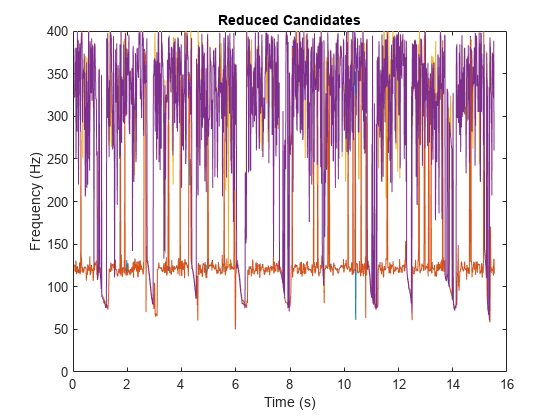### 4. Make Distinctive

If two or more candidates are within a given 5 Hz span, set the candidates to their mean and sum their confidence.

```span = 5; confidenceFactor = 1; for r = 1:size(candidates,1) for c = 1:size(candidates,2) tf = abs(candidates(r,c)-candidates(r,:)) < span; candidates(r,c) = mean(candidates(r,tf)); confidence(r,c) = sum(confidence(r,tf))*confidenceFactor; end end candidates = max(min(candidates,400),50);```

### 5. Forward Iteration of HMM with Octave Smoothing

Now that the candidates have been reduced, you can feed them into an HMM to enforce continuity constraints. Pitch contours are generally continuous for speech signals when analyzed in 10 ms hops. The probability of a pitch moving from one state to another across time is referred to as the emission probability. Emission probabilities can be encapsulated into a matrix which describes the probability of going from any pitch value in a set range to any other in a set range. The emission matrix used in this example was created using the Graz database. 

Load the emission matrix and associated range. Plot the probability density function (PDF) of a pitch in 150 Hz state.

```load EmissionMatrix.mat emissionMatrix emissionMatrixRange currentState =150; figure(7) plot(emissionMatrixRange(1):emissionMatrixRange(2),emissionMatrix(currentState - emissionMatrixRange(1)-1,:)) title("Emission PDF for " + currentState + " Hz") xlabel("Next Pitch Value (Hz)") ylabel("Probability")```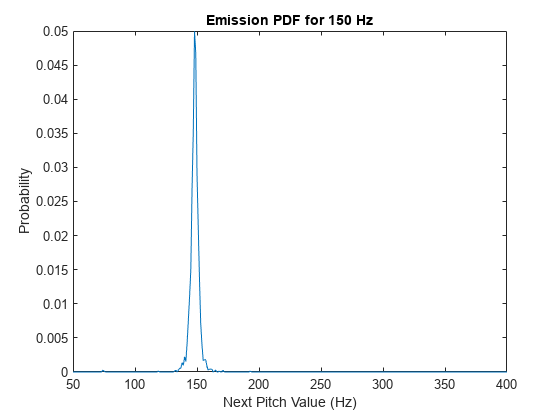The HMM used in this example combines the emission probabilities, which enforce continuity, and the relative confidence of the pitch. At each hop, the emission probabilities are combined with the relative confidence to create a confidence matrix. A best choice for each path is determined as the max of the confidence matrix. The HMM used in this example also assumes that only one path can be assigned to a given state (an assumption of the Viterbi algorithm).In addition to the HMM, this example monitors for octave jumps relative to the short-term median of the pitch paths. If an octave jump is detected, then the backup pitch candidates are added as options for the HMM.

```% Preallocation numPaths = 4; numHops = size(candidates,1); logbook = zeros(numHops,3,numPaths); suspectHops = zeros(numHops,1); % Forward iteration with octave-smoothing for hopNumber = 1:numHops nowCandidates = candidates(hopNumber,:); nowConfidence = confidence(hopNumber,:); % Remove octave jumps if hopNumber > 100 numCandidates = numel(nowCandidates); % Weighted short term median medianWindowLength = 50; aTemp = logbook(max(hopNumber-min(hopNumber,medianWindowLength),1):hopNumber-1,1,:); shortTermMedian = median(aTemp(:)); previousM = mean([longTermMedian,shortTermMedian]); lowerTight = max((4/3)*previousM,emissionMatrixRange(1)); upperTight = min((2/3)*previousM,emissionMatrixRange(2)); numCandidateOutside = sum([nowCandidates < lowerTight, nowCandidates > upperTight]); % If at least 1 candidate is outside the octave centered on the % short-term median, add the backup pitch candidates that were % generated by the normalized correlation function and cepstrum % pitch determination algorithms as potential candidates. if numCandidateOutside > 0 % Apply the backup pitch estimators nowCandidates = [nowCandidates,BackupCandidates(hopNumber,:)];%#ok<AGROW> nowConfidence = [nowConfidence,repmat(mean(nowConfidence),1,2)];%#ok<AGROW> % Make distinctive span = 10; confidenceFactor = 1.2; for r = 1:size(nowCandidates,1) for c = 1:size(nowCandidates,2) tf = abs(nowCandidates(r,c)-nowCandidates(r,:)) < span; nowCandidates(r,c) = mean(nowCandidates(r,tf)); nowConfidence(r,c) = sum(nowConfidence(r,tf))*confidenceFactor; end end end end % Create confidence matrix confidenceMatrix = zeros(numel(nowCandidates),size(logbook,3)); for pageIdx = 1:size(logbook,3) if hopNumber ~= 1 pastPitch = floor(logbook(hopNumber-1,1,pageIdx)) - emissionMatrixRange(1) + 1; else pastPitch = nan; end for candidateNumber = 1:numel(nowCandidates) if hopNumber ~= 1 % Get the current pitch and convert to an index in the range currentPitch = floor(nowCandidates(candidateNumber)) - emissionMatrixRange(1) + 1; confidenceMatrix(candidateNumber,pageIdx) = ... emissionMatrix(currentPitch,pastPitch)*logbook(hopNumber-1,2,pageIdx)*nowConfidence(candidateNumber); else confidenceMatrix(candidateNumber,pageIdx) = 1; end end end % Assign an estimate for each path for pageIdx = 1:size(logbook,3) % Determine most confident transition from past to current pitch [~,idx] = max(confidenceMatrix(:)); % Convert confidence matrix index to pitch and logbook page [chosenPitch,pastPitchIdx] = ind2sub([numel(nowCandidates),size(logbook,3)],idx); logbook(hopNumber,:,pageIdx) = ... [nowCandidates(chosenPitch), ... confidenceMatrix(chosenPitch,pastPitchIdx), ... pastPitchIdx]; % Remove the chosen current pitch from the confidence matrix confidenceMatrix(chosenPitch,:) = NaN; end % Normalize confidence logbook(hopNumber,2,:) = logbook(hopNumber,2,:)/sum(logbook(hopNumber,2,:)); end```

### 6. Traceback of HMM

Once the forward iteration of the HMM is complete, the final pitch contour is chosen as the most confident path. Walk backward through the log book to determine the pitch contour output by the HMM. Calculate the GPE and plot the new pitch contour and the known contour.

```numHops = size(logbook,1); keepLooking = true; index = numHops + 1; while keepLooking index = index - 1; if abs(max(logbook(index,2,:))-min(logbook(index,2,:)))~=0 keepLooking = false; end end [~,bestPathIdx] = max(logbook(index,2,:)); bestIndices = zeros(numHops,1); bestIndices(index) = bestPathIdx; for ii = index:-1:2 bestIndices(ii-1) = logbook(ii,3,bestIndices(ii)); end bestIndices(bestIndices==0) = 1; f0 = zeros(numHops,1); for ii = (numHops):-1:2 f0(ii) = logbook(ii,1,bestIndices(ii)); end f0toPlot = f0; f0toPlot(~isVoiced) = NaN; GPE = mean( abs(f0toPlot(isVoiced) - truePitch(isVoiced)) > truePitch(isVoiced).*p).*100; figure(8) plot(t0,[truePitch,f0toPlot]) legend("Reference","Estimate") ylabel("F0 (Hz)") xlabel("Time (s)") title("GPE = " + round(GPE,2) + " (%)")```### 7. Moving Median Filter

As a final post-processing step, apply a moving median filter with a window length of three hops. Calculate the final GPE and plot the final pitch contour and the known contour.

```f0 = movmedian(f0,3); f0(~isVoiced) = NaN; GPE = mean(abs(f0(isVoiced) - truePitch(isVoiced)) > truePitch(isVoiced).*p).*100; figure(9) plot(t0,[truePitch,f0]) legend("Reference","Estimate") ylabel("F0 (Hz)") xlabel("Time (s)") title("GPE = " + round(GPE,2) + " (%)")```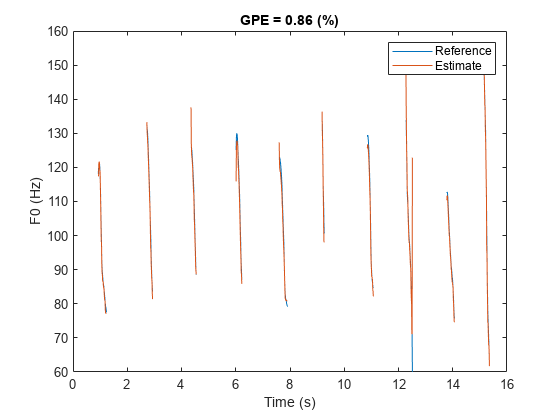### Performance Evaluation

The `HelperPitchTracker` function uses an HMM to apply continuity constraints to pitch contours. The emission matrix of the HMM can be set directly. It is best to train the emission matrix on sound sources similar to the ones you want to track.

This example uses the Pitch Tracking Database from Graz University of Technology (PTDB-TUG) . The data set consists of 20 English native speakers reading 2342 phonetically rich sentences from the TIMIT corpus. Download and extract the data set.

```downloadFolder = matlab.internal.examples.downloadSupportFile("audio","ptdb-tug.zip"); dataFolder = tempdir; unzip(downloadFolder,dataFolder) dataset = fullfile(dataFolder,"ptdb-tug");```

Create an audio datastore that points to the microphone recordings in the database. Set the label associated with each file to the location of the associated known pitch file. The dataset contains recordings of 10 female and 10 male speakers. Use `subset` to isolate the 10th female and male speakers. Train an emission matrix based on the reference pitch contours for both male and female speakers 1 through 9.

```ads = audioDatastore([fullfile(dataset,"SPEECH DATA","FEMALE","MIC"),fullfile(dataset,"SPEECH DATA","MALE","MIC")], ... IncludeSubfolders=true, ... FileExtensions=".wav"); wavFileNames = ads.Files; ads.Labels = replace(wavFileNames,["MIC","mic","wav"],["REF","ref","f0"]); idxToRemove = contains(ads.Files,["F10","M10"]); ads1 = subset(ads,idxToRemove); ads9 = subset(ads,~idxToRemove);```

Shuffle the audio datastores.

```ads1 = shuffle(ads1); ads9 = shuffle(ads9);```

The emission matrix describes the probability of going from one pitch state to another. In the following step, you create an emission matrix based on speakers 1 through 9 for both male and female. The database stores reference pitch values, short-term energy, and additional information in the text files with files extension `f0`. The `getReferencePitch` function reads in the pitch values if the short-term energy is above a threshold. The threshold was determined empirically in listening tests. The `HelperUpdateEmissionMatrix` creates a 2-dimensional histogram based on the current pitch state and the next pitch state. After the histogram is created, it is normalized to create an emission matrix.

```emissionMatrixRange = [50,400]; emissionMatrix = []; for i = 1:numel(ads9.Files) x = getReferencePitch(ads9.Labels{i}); emissionMatrix = HelperUpdateEmissionMatrix(x,emissionMatrixRange,emissionMatrix); end emissionMatrix = emissionMatrix + sqrt(eps); emissionMatrix = emissionMatrix./norm(emissionMatrix);```

Define different types of background noise: white, ambiance, engine, jet, and street. Resample them to 16 kHz to help speed up testing the database.

Define the SNR to test, in dB, as 10, 5, 0, -5, and -10.

```noiseType = ["white","ambiance","engine","jet","street"]; numNoiseToTest = numel(noiseType); desiredFs = 16e3; whiteNoiseMaker = dsp.ColoredNoise(Color="white",SamplesPerFrame=40000,RandomStream="mt19937ar with seed",BoundedOutput=true); noise{1} = whiteNoiseMaker(); [ambiance,ambianceFs] = audioread("Ambiance-16-44p1-mono-12secs.wav"); noise{2} = resample(ambiance,desiredFs,ambianceFs); [engine,engineFs] = audioread("Engine-16-44p1-stereo-20sec.wav"); noise{3} = resample(engine,desiredFs,engineFs); [jet,jetFs] = audioread("JetAirplane-16-11p025-mono-16secs.wav"); noise{4} = resample(jet,desiredFs,jetFs); [street,streetFs] = audioread("MainStreetOne-16-16-mono-12secs.wav"); noise{5} = resample(street,desiredFs,streetFs); snrToTest = [10,5,0,-5,-10]; numSNRtoTest = numel(snrToTest);```

Run the pitch detection algorithm for each SNR and noise type for each file. Calculate the average GPE across speech files. This example compares performance with the popular pitch tracking algorithm: Sawtooth Waveform Inspired Pitch Estimator (SWIPE). A MATLAB® implementation of the algorithm can be found at . To run this example without comparing to other algorithms, set `compare` to `false`. The following comparison takes around 15 minutes.

```compare =true; numFilesToTest = 20; p = 0.1; GPE_pitchTracker = zeros(numSNRtoTest,numNoiseToTest,numFilesToTest); if compare GPE_swipe = GPE_pitchTracker; end for i = 1:numFilesToTest [cleanSpeech,info] = read(ads1); cleanSpeech = resample(cleanSpeech,desiredFs,info.SampleRate); truePitch = getReferencePitch(info.Label{:}); isVoiced = truePitch~=0; truePitchInVoicedRegions = truePitch(isVoiced); for j = 1:numSNRtoTest for k = 1:numNoiseToTest noisySpeech = mixSNR(cleanSpeech,noise{k},snrToTest(j)); f0 = HelperPitchTracker(noisySpeech,desiredFs,EmissionMatrix=emissionMatrix,EmissionMatrixRange=emissionMatrixRange); f0 = [0;f0]; % manual alignment with database. GPE_pitchTracker(j,k,i) = mean(abs(f0(isVoiced) - truePitchInVoicedRegions) > truePitchInVoicedRegions.*p).*100; if compare f0 = swipep(noisySpeech,desiredFs,[50,400],0.01); f0 = f0(3:end); % manual alignment with database. GPE_swipe(j,k,i) = mean(abs(f0(isVoiced) - truePitchInVoicedRegions) > truePitchInVoicedRegions.*p).*100; end end end end GPE_pitchTracker = mean(GPE_pitchTracker,3); if compare GPE_swipe = mean(GPE_swipe,3); end```

Plot the gross pitch error for each noise type.

```for ii = 1:numel(noise) figure plot(snrToTest,GPE_pitchTracker(:,ii),"b") hold on if compare plot(snrToTest,GPE_swipe(:,ii),"g") end plot(snrToTest,GPE_pitchTracker(:,ii),"bo") if compare plot(snrToTest,GPE_swipe(:,ii),"gv") end title(noiseType(ii)) xlabel("SNR (dB)") ylabel("Gross Pitch Error (p = " + round(p,2) + " )") if compare legend("HelperPitchTracker","SWIPE") else legend("HelperPitchTracker") end grid on hold off end```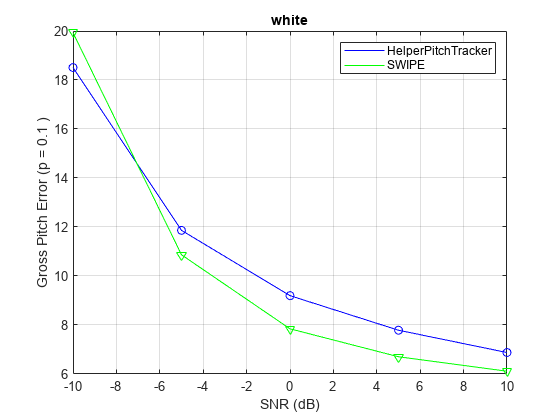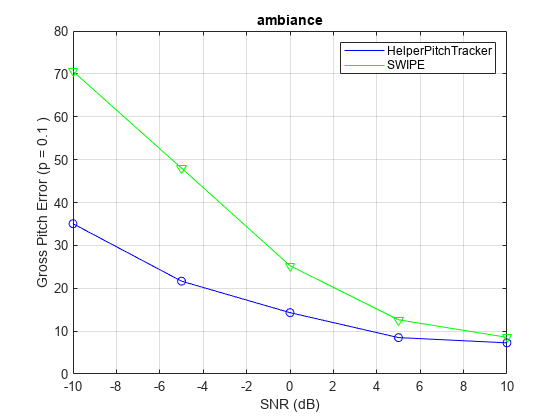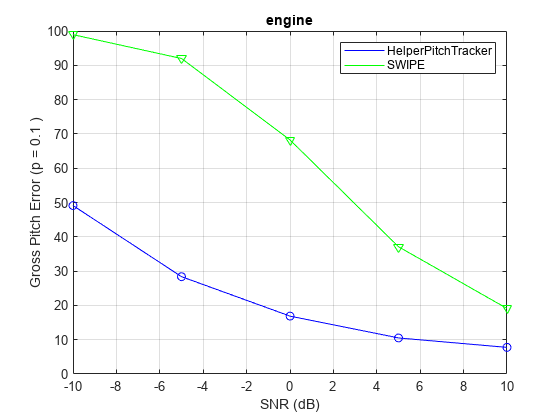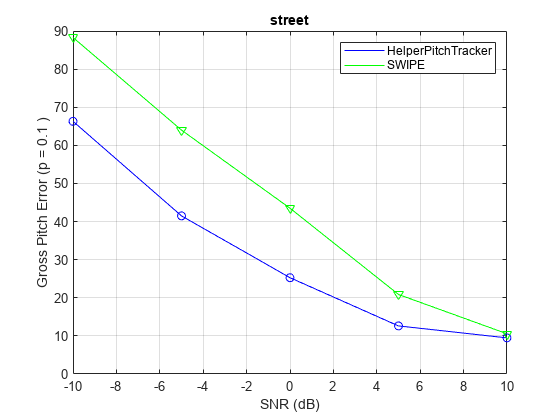### Conclusion

You can use `HelperPitchTracker` as a baseline for evaluating GPE performance of your pitch tracking system, or adapt this example to your application.

### References

 G. Pirker, M. Wohlmayr, S. Petrik, and F. Pernkopf, "A Pitch Tracking Corpus with Evaluation on Multipitch Tracking Scenario", Interspeech, pp. 1509-1512, 2011.

 Drugman, Thomas, and Abeer Alwan. "Joint Robust Voicing Detection and Pitch Estimation Based on Residual Harmonics." Proceedings of the Annual Conference of the International Speech Communication Association, INTERSPEECH. 2011, pp. 1973-1976.

 Gonzalez, Sira, and Mike Brookes. "A Pitch Estimation Filter robust to high levels of noise (PEFAC)." 19th European Signal Processing Conference. Barcelona, 2011, pp. 451-455.

 Signal Processing and Speech Communication Laboratory. Accessed September 26, 2018. https://www.spsc.tugraz.at/databases-and-tools/ptdb-tug-pitch-tracking-database-from-graz-university-of-technology.html.

 "Arturo Camacho." Accessed September 26, 2018. https://www.cise.ufl.edu/~acamacho/english/.

 "Fxpefac." Description of Fxpefac. Accessed September 26, 2018. http://www.ee.ic.ac.uk/hp/staff/dmb/voicebox/voicebox.html.